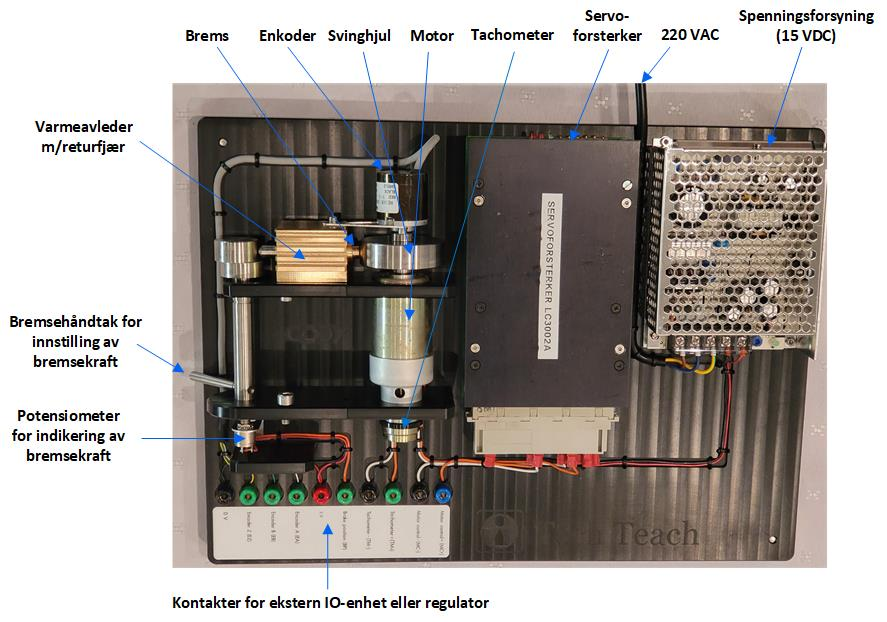# DC-motor

Figure 1 shows a DC motor (Faulhaber) including:

·         a speed sensor in the form of a tachometer, which gives a voltage proportional to the rotational speed,

·         a servo amplifier,

·         a power supply.

The control signal is in the range of ±10V, and the tachometer voltage is in the range of approximately ±10V, corresponding to a speed of ± 2krpm. A load inertia has been added to the motor. The time constant of the motor including load and tachometer is approximately 0.3s. The gain from control signal [V] to tachometer measurement [V] is approximately 0.85.Figure 1

### Mathematical model

Various mathematical models may represent the motor more or less accurately, e.g.

- A differential equation model representing “time-constant dynamics”:

T*dS/dt + S = K*(C + L)       (1)

where S [krpm] is the speed. C [V] is the control signal. L [V] is an equivalent voltage representing the load torque. L is in the range of ± 10 V. Approximate values of the model parameters are: K = 0.17 krpm/V, T = 0.3 s.

For the tachometer, the following relation between the speed measurement Sm [V] and the speed S [krpm] can be assumed:

Sm = KmS

where Km = 5 V/krpm.

### Experimental data

logfile1.txt is a text file containing time, control signal, and speed measurements as tachometer voltage from an experiment where the control signal was adjusted manually.

Updated 18. March 2018. Finn Aakre Haugen (finn@techteach.no).